Next: Mechanical interaction between macrosystems Up: Statistical thermodynamics Previous: Thermal interaction between macrosystems

## Temperature

Suppose that the systemsand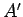are initially thermally isolated from one another, with respective energies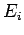and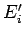. (Since the energy of an isolated system cannot fluctuate, we do not have to bother with mean energies here.) If the two systems are subsequently placed in thermal contact, so that they are free to exchange heat energy, then, in general, the resulting state is an extremely improbable one [i.e.,is much less than the peak probability]. The configuration will, therefore, tend to change in time until the two systems attain final mean energies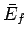and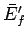which are such that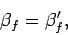(176)

whereand. This corresponds to the state of maximum probability (see Sect. 5.2). In the special case where the initial energies,and, lie very close to the final mean energies,and, respectively, there is no change in the two systems when they are brought into thermal contact, since the initial state already corresponds to a state of maximum probability.

It follows from energy conservation that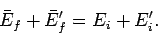(177)

The mean energy change in each system is simply the net heat absorbed, so that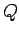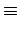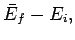(178)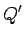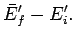(179)

The conservation of energy then reduces to(180)

i.e., the heat given off by one system is equal to the heat absorbed by the other (in our notation absorbed heat is positive and emitted heat is negative).

It is clear that if the systemsandare suddenly brought into thermal contact then they will only exchange heat and evolve towards a new equilibrium state if the final state is more probable than the initial one. In other words, if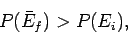(181)

or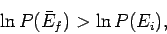(182)

since the logarithm is a monotonic function. The above inequality can be written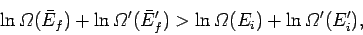(183)

with the aid of Eq. (159). Taylor expansion to first order yields(184)

which finally gives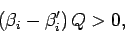(185)

where,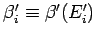, and use has been made of Eq. (180).

It is clear, from the above, that the parameter, defined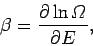(186)

has the following properties:
1. If two systems separately in equilibrium have the same value ofthen the systems will remain in equilibrium when brought into thermal contact with one another.
2. If two systems separately in equilibrium have different values ofthen the systems will not remain in equilibrium when brought into thermal contact with one another. Instead, the system with the higher value ofwill absorb heat from the other system until the twovalues are the same [see Eq. (185)].
Incidentally, a partial derivative is used in Eq. (186) because in a purely thermal interaction the external parameters of the system are held constant whilst the energy changes.

Let us define the dimensionless parameter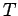, such that(187)

whereis a positive constant having the dimensions of energy. The parameteris termed the thermodynamic temperature, and controls heat flow in much the same manner as a conventional temperature. Thus, if two isolated systems in equilibrium possess the same thermodynamic temperature then they will remain in equilibrium when brought into thermal contact. However, if the two systems have different thermodynamic temperatures then heat will flow from the system with the higher temperature (i.e., the hotter'' system) to the system with the lower temperature until the temperatures of the two systems are the same. In addition, suppose that we have three systems,, and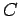. We know that ifandremain in equilibrium when brought into thermal contact then their temperatures are the same, so that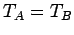. Similarly, ifandremain in equilibrium when brought into thermal contact, then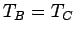. But, we can then conclude that, so systemsandwill also remain in equilibrium when brought into thermal contact. Thus, we arrive at the following statement, which is sometimes called the zeroth law of thermodynamics:
If two systems are separately in thermal equilibrium with a third system then they must also be in thermal equilibrium with one another.

The thermodynamic temperature of a macroscopic body, as defined in Eq. (187), depends only on the rate of change of the number of accessible microstates with the total energy. Thus, it is possible to define a thermodynamic temperature for systems with radically different microscopic structures (e.g., matter and radiation). The thermodynamic, or absolute, scale of temperature is measured in degrees kelvin. The parameteris chosen to make this temperature scale accord as much as possible with more conventional temperature scales. The choice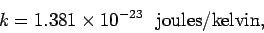(188)

ensures that there are 100 degrees kelvin between the freezing and boiling points of water at atmospheric pressure (the two temperatures are 273.15 and 373.15 degrees kelvin, respectively). The above number is known as the Boltzmann constant. In fact, the Boltzmann constant is fixed by international convention so as to make the triple point of water (i.e., the unique temperature at which the three phases of water co-exist in thermal equilibrium) exactly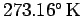. Note that the zero of the thermodynamic scale, the so called absolute zero of temperature, does not correspond to the freezing point of water, but to some far more physically significant temperature which we shall discuss presently.

The familiar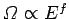scaling for translational degrees of freedom yields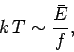(189)

using Eq. (187), sois a rough measure of the mean energy associated with each degree of freedom in the system. In fact, for a classical system (i.e., one in which quantum effects are unimportant) it is possible to show that the mean energy associated with each degree of freedom is exactly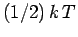. This result, which is known as the equipartition theorem, will be discussed in more detail later on in this course.

The absolute temperatureis usually positive, since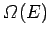is ordinarily a very rapidly increasing function of energy. In fact, this is the case for all conventional systems where the kinetic energy of the particles is taken into account, because there is no upper bound on the possible energy of the system, andconsequently increases roughly like. It is, however, possible to envisage a situation in which we ignore the translational degrees of freedom of a system, and concentrate only on its spin degrees of freedom. In this case, there is an upper bound to the possible energy of the system (i.e., all spins lined up anti-parallel to an applied magnetic field). Consequently, the total number of states available to the system is finite. In this situation, the density of spin states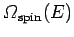first increases with increasing energy, as in conventional systems, but then reaches a maximum and decreases again. Thus, it is possible to get absolute spin temperatures which are negative, as well as positive.

In Lavoisier's calorific theory, the basic mechanism which forces heat to flow from hot to cold bodies is the supposed mutual repulsion of the constituent particles of calorific fluid. In statistical mechanics, the explanation is far less contrived. Heat flow occurs because statistical systems tend to evolve towards their most probable states, subject to the imposed physical constraints. When two bodies at different temperatures are suddenly placed in thermal contact, the initial state corresponds to a spectacularly improbable state of the overall system. For systems containing of order 1 mole of particles, the only reasonably probable final equilibrium states are such that the two bodies differ in temperature by less than 1 part in. The evolution of the system towards these final states (i.e., towards thermal equilibrium) is effectively driven by probability.Next: Mechanical interaction between macrosystems Up: Statistical thermodynamics Previous: Thermal interaction between macrosystems
Richard Fitzpatrick 2006-02-02